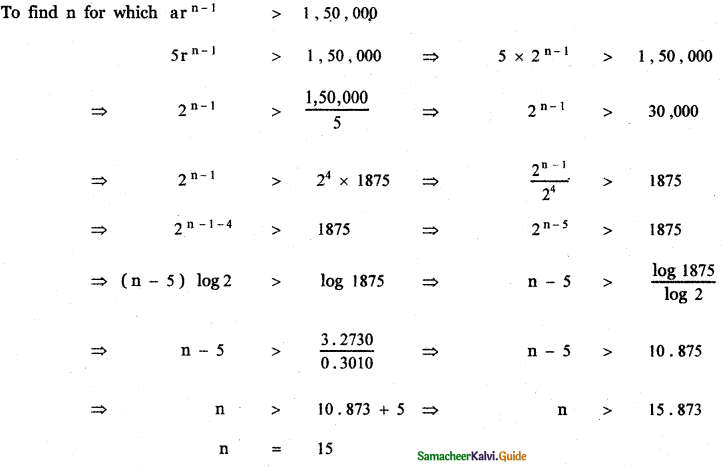Tamilnadu State Board New Syllabus Samacheer Kalvi 11th Maths Guide Pdf Chapter 5 Binomial Theorem, Sequences and Series Ex 5.3 Text Book Back Questions and Answers, Notes.

## Tamilnadu Samacheer Kalvi 11th Maths Solutions Chapter 5 Binomial Theorem, Sequences and Series Ex 5.3Question 1.
Find the sum of the first 20 terms of the arithmetic progression having the sum of first 10 terms as 52 and the sum of the first 15 terms as 77 .
Sum of the first n terms of an Arithmetic progression is Sn =  [2a + (n – 1)d]
Given S10 = 52
52 = $$\frac{10}{2}$$ [ 2a + (10 – 1) d ]
52 = 5 [2a + 9d]
52 = 10a + 45d ……….. (1)
Also given S15 = 77
77 = $$\frac{15}{2}$$ [2a + (15 – 1)d]
77 = $$\frac{15}{2}$$ [2a + 14d]
77 = 15 [a + 7d]
77 = 15a + 105d ………….. (2)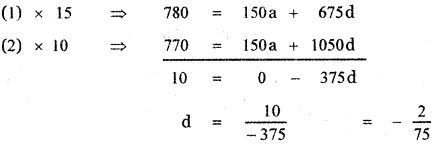Substituting the value of d in (1)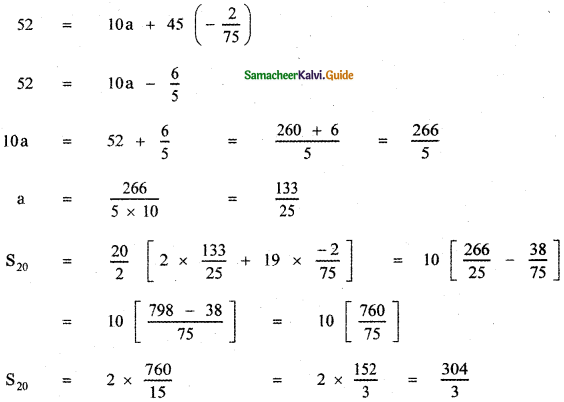Question 2.
Find the sum up to the 17th term of the series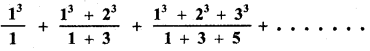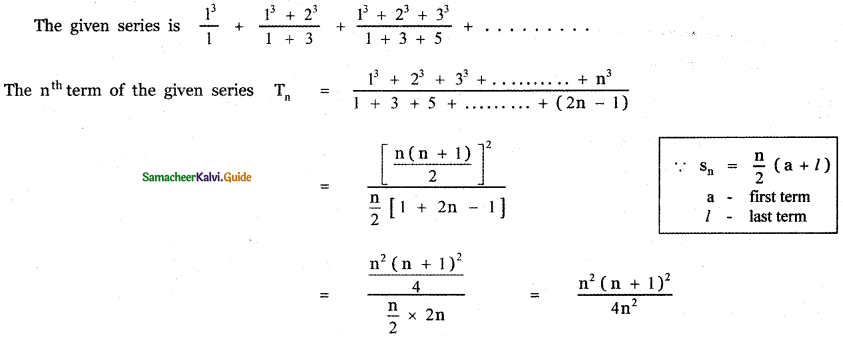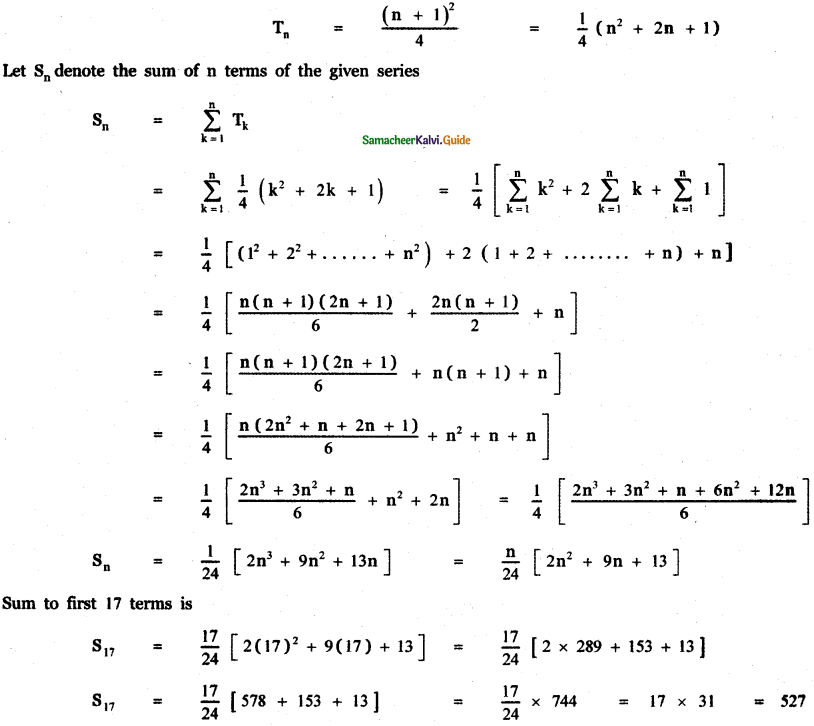Question 3.
Compute the sum of first n terms of the following series.
(i) 8 + 88 + 888 + 8888 + . . . . . . .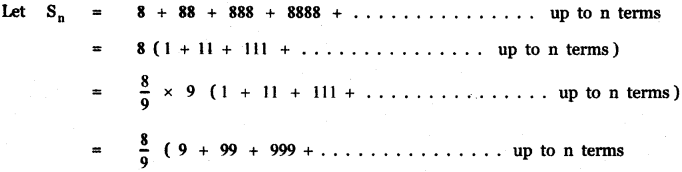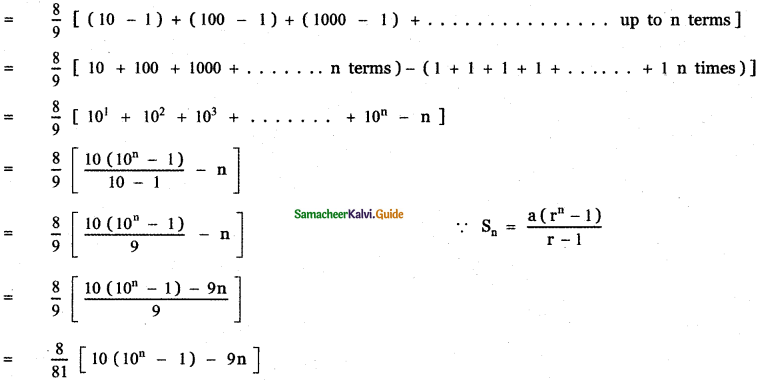(ii) 6 + 66 + 666 + 6666 + . . . . . . .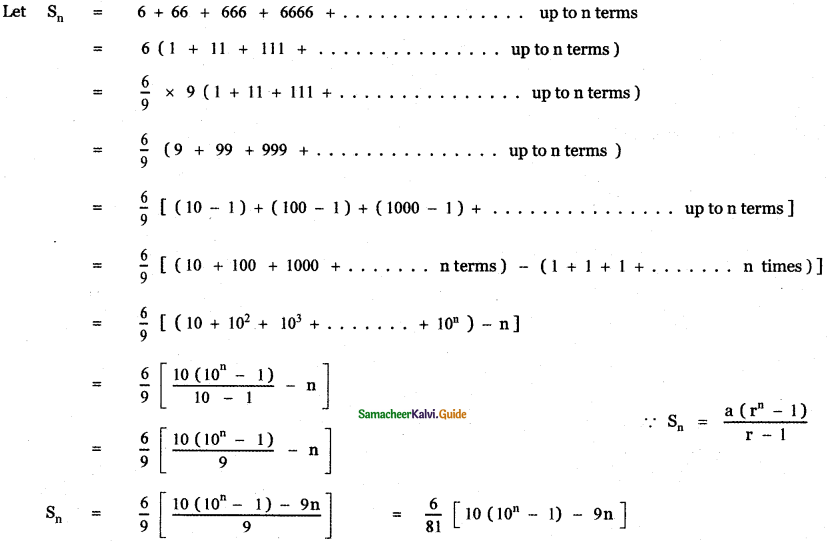Question 4.
Compute the sum of first n terms of 1 + (1 + 4) + (1 + 4 + 42) + (1 + 4 + 42 + 43) + …………..
The given series is 1 + (1 + 4) + (1 + 4 + 42) + (1 + 4 + 42 + 43) + …………..
nth term of the series is Tn = 1 + 4 + 42 + 43 + ………………. + 4n-1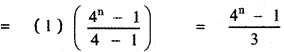Sum to n terms of the series
Sn = T1 + T2 + ………….. + Tn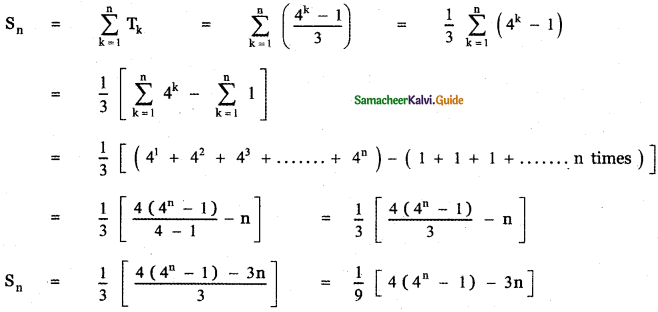Question 5.
Find the general terms and sum to n terms of the sequence 1, $$\frac{4}{3}$$, $$\frac{7}{9}$$, $$\frac{10}{27}$$, ………….
The given sequence as 1, $$\frac{4}{3}$$, $$\frac{7}{9}$$, $$\frac{10}{27}$$, ………….
Consider the terms in the numerator 1, 4, 7, 10, ……………….. which is an Arithmetic progression
with first term a = 1, common difference d = 4 – 1 = 3
an = a + (n – 1)d = 1 + (n – 1)3 = 1 + 3n – 3 = 3n – 2
The given sequence can be written as 1, (1 + 3)$$\left(\frac{1}{3}\right)$$, (1 + 2 × 3)$$\left(\frac{1}{3}\right)^{2}$$ (1 + 3 × 3) $$\left(\frac{1}{3}\right)^{3}$$, …………….
where $$1, \frac{1}{3}, \frac{1}{3^{2}}, \frac{1}{3^{3}}, \ldots \ldots$$ is a G. P with first term a = 1 , common ratio r = $$\frac{1}{3}$$
∴ The given sequence is an Arithmetico – Geometric sequence.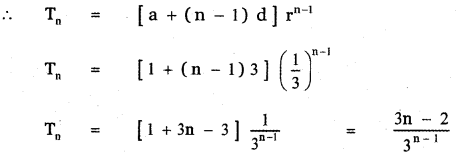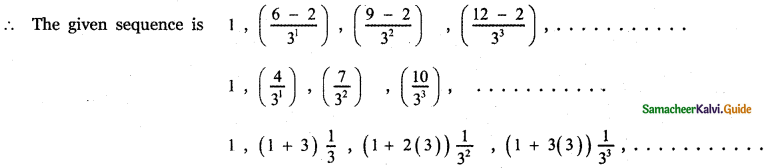which is an arithmetic – geometric sequence.
∴ The sum of first n terms of the arithmetico – geometric sequence is given by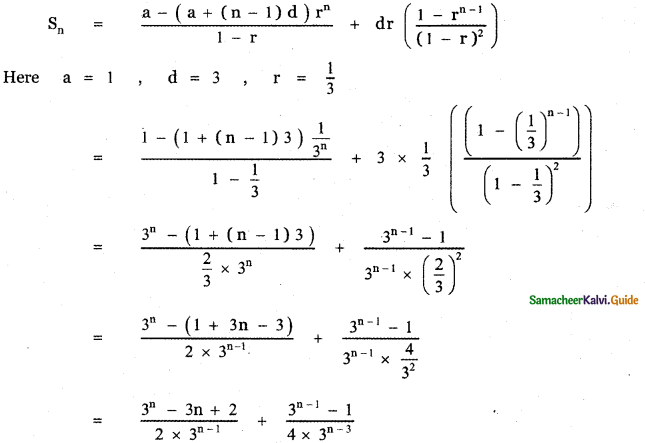Question 6.
Find the value of n if the sum to n terms of the series $$\sqrt{3}+\sqrt{75}+\sqrt{243}+\ldots \ldots . . . \text { is } 435 \sqrt{3}$$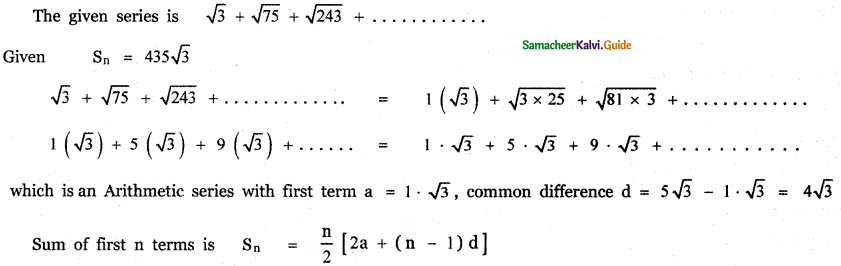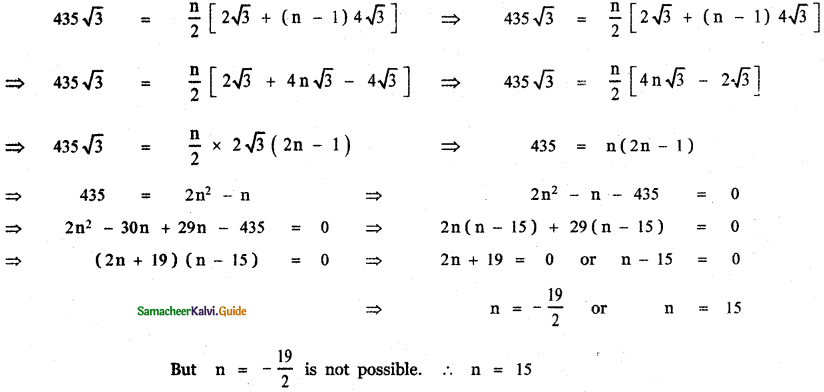Question 7.
Show that the sum of ( m + n)th and ( m – n)th term of an A.P is equal to twice the mth term.
Let the A.P. be a, a + d, a + 2d, ……..
tm + n = a + (m + n – 1)d
tm – n = a + (m – n – 1)d
tm = a + (m – 1)d
2tm = 2[a + (m – 1)d]
To prove tm + n + tm – n = 2tm
LHS tm + n + tm – n = a + (m + n – 1)d + a + (m – n – 1)d= 2a + d [2m – 2]
= 2[a + (m – 1)d) = 2 tm = RHSQuestion 8.
A man repays an amount of Rs.3250 by paying Rs.20 in the first month and then increases the payment by Rs. 15 per month. How long will it take him to clear the amount?
Amount of loan = 3250
Let n be the number of months taken to clear the loan
Amount paid in the first month a = 20
Increased payment in every month d = 15
∴ Amount paid in the second month = 20 + 15 = 35
Amount paid in the third month = 35 + 15 = 50
∴ The sequence of amount paid in every month is 20, 35, 50, …………. which is an A.P with first term a = 20 and common difference = 15
Given Sn = $$\frac{n}{2}$$ [2a + (n – 1) d]
Sn = 3250
3250 = $$\frac{n}{2}$$ [2 × 20 + (n – 1) 15]
6500 = n[40 + 15n – 15]
6500 = n[25 + 15n]
6500 = 25n + 15n2
1300 = 5n + 3n2
3n2 + 5n – 1300 = 0
3n2 + 65n – 60n – 1300 = 0
n(3n + 65) – 20 (3n + 65) = 0
(n – 20) (3n + 65) = 0
n = 20 = 0 or 3n + 65 = 0
n = 20 or n = $$-\frac{65}{3}$$
n = $$-\frac{65}{3}$$ is not possible ∴ n = 20
Thus, in 20 months the loan is cleared.Question 9.
In a race, 20 balls are placed in a line at intervals of 4 meters with the first ball, 24 meters away from the starting point. A contestant is required to bring the balls back to the starting place one at a time. How far would the contestant run to bring back all balls?
Number of balls placed in a line = 20
Let A be the starting point.
The distance of the first ball from A = 24 m
The distance of the second ball from the first ball = 4 m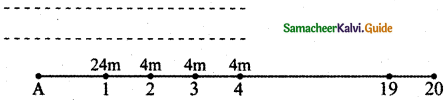A contestant starts from point A, travels a distance of 24 m and picks the first ball and brings it back to starting point A. This is continued for each ball.
Distance travelled by the contestant to bring the first ball = 24 + 24 = 2 × 24 = 48 m
Distance travelled to bring the second ball = 2 ( 24 + 4 ) = 2 × 28 = 56 m
Distance travelled to bring the third ball = 2 × (24 + 4 + 4) = 64 m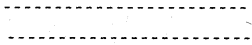∴ The sequence of distances travelled are 48, 56, 64 ……………
This is an Arithmetic progression with first term a = 48 ,
common difference d = 56 – 48 = 8 ,
number of terms n = 20.
The sum of 20 terms of this A.P gives the total distance travelled by the contestant in bringing all balls to the starting place
Sn = $$\frac{\mathrm{n}}{2}$$ [2a + (n – 1)d]
S20 = $$\frac{\mathrm{20}}{2}$$ [2 × 48 + (20 – 1)8] = 10 [96 + 19 × 8]
= 10 [ 96 + 152] = 10 × 248 = 2480
Total distance traveled = 2480 m

Question 10.
The number of bacteria in a certain culture doubles every hour. If there were 30 bacteria present in the culture originally how many bacteria will be present at the end of the 2nd hour, 4th hour, and nth hour?
Number of bacteria at the beginning = 30
Number of bacteria after 1 hour = 30 × 2 = 60
Number of bacteria after 2 hours = 30 × 22 = 120
Number of bacteria after 4 hours = 30 × 24 = 30 × 16 = 480
∴ Number of bacteria after nth hour = 30 × 2nQuestion 11.
What will Rs. 500 amounts to in 10 years after its deposit in a bank which pays an annual interest rate of 10 % compounded annually?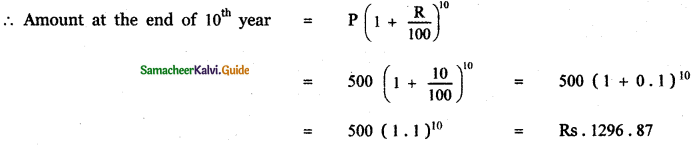∴ The sequence of a number of viruses in each day is 5, 10, 20, 40, 80, ………………. which is a G. P with First-term a = 5, Common ratio r = $$\frac{10}{5}$$ = 2